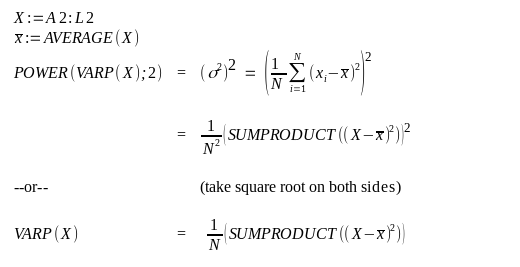# Why these two formulas are not exactly the same

I have 2 formulas which should give the same number but they differ by about 1%:

`=POWER(VARP(A2:L2);2)` gives 142,006944
but

`=(SUMPRODUCT((A2:L2-6,5)^2))` gives 143

Here 6,5 is the `=AVERAGE(A2:L2)`

Please see the two snippets below.

-1- Please stop posting such images. Lots of “area”, very little information. Attach a sample document instead if not the question is explicitly about the view.
-2- Why would you expect equal results? `11.91667 * 12 <> 11.91667^2` should be obvious.

I do not follow your answer. I do not know why it matters posting pictures of my problem, are you using a mobile phone that such a reasonably small area matters? Also, both these formulas should be the `second central moment` and hence the same.

You need to compare `VARP(A2:L2)` and `=SUMPRODUCT((A2:L2-6,5)^2)/COUNT(A2:L2)` (Average of deviation squares).

To make it slightly clearer: why do you square variance? There you have your error. Both @Lupp and @anon73440385 have mentioned that.

@mikekaganski - here seems to be the trap caused by standard nomenclature of variance:=σ2 and assuming function `VARP()` calculates σ.

This is strange: `=POWER(SUMPRODUCT(((A2:L2-6,5)^2)/12);2)` computes the `=VARP` but I’m confused why there is `=POWER....; 2)` ?? The `variance =VARP` should be `E(X-EX)^2` which is exactly
`=SUMPRODUCT((A2:L2-6,5)^2)/COUNT(A2:L2)` but only after `=POWER....; 2)` this is equal to `=VARP`: in LibreOffuice, why there must be this extra `=POWER`? for equality with `=VARP`?

`=POWER(SUMPRODUCT(((A2:L2-6,5)^2)/12);2)` computes the `=VARP`

No.

@anon73440385: quite true!

I do not know why it matters posting pictures of my problem, are you using a mobile phone that such a reasonably small area matters?

It is not bad to post screenshots of your problem per se. However, it is good when poster only posts screenshots in addition to also posting the editable document, so that others do not waste time re-creating sample documents to experiment with, possibly also being uncertain if the screenshot had hidden some important details like numeric precision defined in number format of the cells in question. Yes, it’s rather easy to reconstruct this sample anew, but even this case would be more friendly to those who you intend to get help from, if you still provide the sample.

Hello,

I did not intend to add an answer to this but due to lengthy discussion, revisited my decision.The short version of the answer to your question: The 2 formulas calculate different values and are not equal.

For a more detailed answer, check this image of pseudo formulas:why there must be this extra `=POWER`

It was you, who started using this. No comment stated that it is required, but all comments tried to convince you of the contrary. Therefore the only reason to start the formulas above with `POWER()` as well, is motivated by trying to clarify the original, underlying misunderstanding.